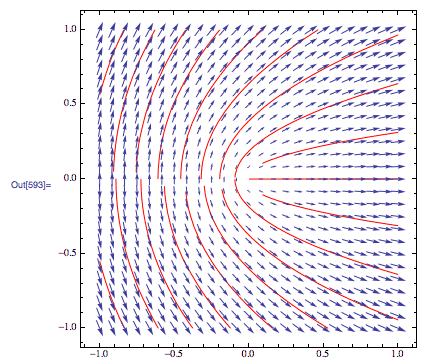# How to find the constant in this indefinite integration?

Homework Statement:
Find constant of a indefinite integration for classical mechanics
Relevant Equations:
$\int$
$$x(t)=\int \dot{x}(t)\mathrm dt=vt+c$$

That's what I did. But, book says

$$x(t)=\int \dot{x}(t)\mathrm dt=x_0+v_0 t+ \frac{F_0}{2m}t^2$$

Seems like, $$x_0 + \dfrac{a_0}{2}t^2$$ is constant. How to find constant is equal to what?

fresh_42
Mentor
To find the value of the constant, you will need a initial condition, often ##x(0)=0## or in general ##x(t_0)=x_0##. Then insert it in your equation and solve for ##c##.

The reason is the following:
A differential equation has - depending on c - many solutions. Each solution corresponds to a flow through a vector field which is defined by the differential equation. The initial condition tells us where to start the flow, such that it will be the unique one that solves the equation plus its initial condition.ref.: https://mathematica.stackexchange.com/questions/22190/why-are-these-flow-lines-cut-short

Doc Al
Mentor
What is ##\dot{x}(t)## as a function of t? Don't assume it's a constant.

To find the value of the constant, you will need a initial condition, often x(0)=0 or in general x(t0)=c0. Then insert it in your equation and solve for c.
If I try to do this way then, I get c=-vt

0=vt+c
c=-vt

Doc Al
Mentor
is velocity... It's obviously constant [as far as I know]
Why do you assume that? Your book didn't!

Why do you assume that? Your book didn't!
What my book didn't do?

Doc Al
Mentor
What my book didn't do?
It looks to me as if the problem assumes a constant acceleration, not a constant velocity.

fresh_42
Mentor
If I try to do this way then, I get c=-vt

0=vt+c
c=-vt
If you set ##t=0## then you get ##c=x(0)## in the first equation, and ##x(0)=x_0## in the second.

Initial conditions could as well be at any time and of any value, e.g. ##x(5)=7## but it is usually ##x(0)## we are interested in because it makes the equations easier to solve for ##c##. It is also where the name comes from: initial as in starting point which is usually ##t=0.##

•Istiakshovon
If you set ##t=0## then you get ##c=x(0)## in the first equation, and ##x(0)=x_0## in the second.

Initial conditions could as well be at any time and of any value, e.g. ##x(5)=7## but it is usually ##x(0)## we are interested in because it makes the equations easier to solve for ##c##. It is also where the name comes from: initial as in starting point which is usually ##t=0.##
So, I can find $$x(t)=vt+x(0)$$ but, my book assumes that $$x(t)=x(0)+vt+\frac{at^2}{2}$$

Doc Al
Mentor
So, I can find $$x(t)=vt+x(0)$$ but, my book assumes that $$x(t)=x(0)+vt+\frac{at^2}{2}$$
You assume that the velocity is constant, but your book does not.

•Delta2
You assume that the velocity is constant, but your book does not.
How? If I integrate velocity than, I get position not acceleration...Doc Al
Mentor
How? If I integrate velocity than, I get position not acceleration...You're trying to find the position.

Do this: Start with a constant acceleration. Then integrate to find the velocity, and then integrate again to find the position.

•Istiakshovon
fresh_42
Mentor
So, I can find $$x(t)=vt+x(0)$$ but, my book assumes that $$x(t)=x(0)+vt+\frac{at^2}{2}$$
So? Your book says ##x(0)=v\cdot 0 +x(0)## in the first and ##x(0)=x(0) + v\cdot 0 +\frac{a\cdot 0^2}{2}=x(0)## in the second equation. But what does it say in the problem statement before ##c## occurs?

Without initial condition ##x(t_0)=vt_0+x_0## you get infinitely many solution from ##\dot x =v## and with initial condition only one solution: ##x(t)=v\cdot t +x_0##.

And in order to solve ##\ddot x = a## you need even two initial conditions because you integrate twice and each integral has a ##c##.

•Istiakshovon
Doc Al
Mentor
I got confused... $$\dot{x}=\int \ddot{x} \mathrm dt=\dot{x} t +c$$
That's a good start. Now solve for that constant. Looks like they assume the velocity is v0 when t = 0.

You're trying to find the position.

Do this: Start with a constant acceleration. Then integrate to find the velocity, and then integrate again to find the position.
Ohh! Thanks... got it....

$$\dot{x} (t)=\int \ddot{x} t dt=\ddot{x}t+c$$
$$\dot{x}(t)=\ddot{x}t+\dot{x}(0)$$
$$x(t)=\int \dot{x} (t) dt$$
$$=\int \ddot{x}t+\dot{x_0} dt$$
$$=\dot{x_0}t+\frac{\ddot{x}}{2}t^2+c$$

Doc Al
Mentor
Ohh! Thanks... got it....

$$\dot{x} (t)=\int \ddot{x} t dt=\ddot{x}t+c$$
$$\dot{x}(t)=\ddot{x}t+\dot{x}(0)$$
$$x(t)=\int \dot{x} (t) dt$$
$$=\int \ddot{x}t+\dot{x_0} dt$$
$$=\dot{x_0}t+\frac{\ddot{x}}{2}t^2+c$$
OK, much better!

And it looks like your book assumed a constant acceleration (because of a constant force) equal to F0/m.

•Istiakshovon
OK, much better!

And it looks like your book assumed a constant acceleration (because of a constant force) equal to F0/m.
Hum..!

I don't understand the confusion. We start with a constant force along the x-axis, ##F_0##. Newton's law says,
$$F=ma=m\frac{d^2x}{dt^2}=F_0$$
$$m\frac{d^2x}{dt^2}=m\frac{d\dot x }{dt}$$
$$\frac{d\dot x }{dt}=\frac{F_0}{m}$$
Assuming ##t_0=0## we integrate
$$\int_{\dot {x}_0}^{\dot x}d\dot {x}'=\int_0^t\frac{F_0}{m}dt'$$
with the primes indicating dummy variables of integration.
$$\dot x - \dot {x}_0=\frac{F_0}{m}t$$
$$\dot {x}_0=v_0$$
$$\dot x=\frac{dx}{dt}$$
$$\frac{dx}{dt}= v_0+\frac{F_0}{m}t$$
Integrate again
$$\int_{x_0}^x dx'=\int_{0}^t (v_0+\frac{F_0}{m}t')dt'$$
$$x=x_0 + v_0t+ \frac{F_0t^2}{2m}$$
Does this help or am I missing something?

haruspex